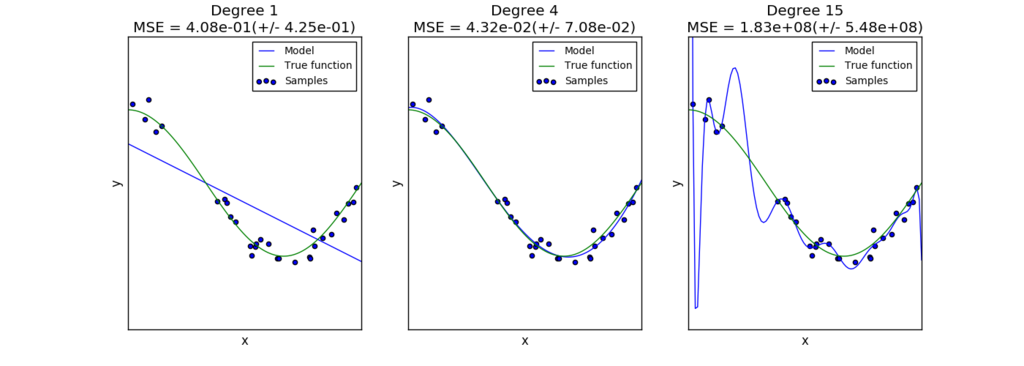Solving the problem of overfitting.

As Cousera's Machine Learning course, I learned regularization to avoid overfitting. There are Underfitting and Overfittng in machine learning. Underfitting refers to a model that can neither model the training data not generalize to new data. This is often not discussed as it is easy to detect given a good performance metric. By contrast, Overfitting refers to a model that models the training data too well. In generally, training data sets includes noise and this noise cause overfitting from effecting model.If we have many features, the learned hypothesis may fit the training set very well (), but fail to generalize to new examples (predict values on new example). We have to generalize how well a hypothesis applies even to new examples in machine learning.

There are two options to avoid overfitting below:

1. Reduce number of features
2. Regularization

Reduce number of features is that you manually select which features to keep and algorithm model. But it means throwing away some of the information that you have data about problem. It is not good a way for solving overfitting. So in generally, Regularization is often used for this problem. Using regularization, you can keep all the features, but reduce magnitude and values of parameters theta. And it works well when you have a lot of features, each of which contributes a bit to predicting y.

Cost Function of Regularization for Overfitting

Regularization gives penalty to cost function to ease a complexity of model. Given some parameters theta, regularization can be simpler hypothesis and less prone to overfitting. We wanna make unimportant parameter that are less likely to be relevant close zero, but we don't know to pick in advance. So, Regularization's formula is below:This regularization means that give penalty to all parameters and make all parameters close zero. There is a trade off between cost function and regularization, cost function's goal is that it would like to fit the training data well but regularization's goal that it want to keep the parameters small. To control this trade off, we useand have to choose optimal value of it because it cause the under fitting if regularization's parameter is too big.

Regularized Linear Regression

In linear regression, we can add regularization to gradient descent with repeat below:So< 1, it suppose around 0.99 which means make parameters a little shrink.

And we can add regularization to normal equation below:But it cause non-invertibility (singular) if, this means that suppose. If, regularization solve this non-invetibility. So you don't need worry about it.